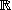!! used as default html header if there is none in the selected theme. OEF derivative

# OEF derivative --- Introduction ---

In this module there are 6 exercices on differentiation .

### The derivative of a combined function

 Given the function : Determine the derivative in

=

NB : write "sqrt(ax+b)" for

### The derivative of a polynomial

 Let be a polynomial , gedefined inby . Let be differentiable in. Determine the derivative.
For all real ,   =

### The product rule

 Determine the derivative of a function indefined by with :
The functions and are differentiable in and :
=
=

In order to determine the derivative of   we apply the following rule of differentiation:

The derivative function of   will be :

=

### The quotient rule

 Given the function defined inby   . We will now determine the derivative of    in a few steps :
• We rewrite as a fraction; ,wherein the functions and are defined inby :
and

• The functions and are differentiable in:
and
• = met :

en

and

• The fraction is differentiable in:
• Which rule on differentiation do we apply :
• is differentiable in.
• Which rule on differentiation do we apply :
• We will get :
=

• ### Tangent and derivative

 Given the plane . The curve C is the graph of the function     , defined in . The line is the tangent of C in point , with coordinates ( : ). Point , with coordinates ( : ) is also on line . Determine the value of     at two decimals accurate. xrange -, yrange -, parallel -,-,-,,1,0, 2*+1, grey parallel -,-,,-,0,1, 2*+1, grey hline 0,0,black vline 0,0,black arrow 0,0,1,0,8, black arrow 0,0,0,1,8, black text black , -0.5,-0.3,small , O text black , 1,-0.3,small , I text black , -0.5,1,small , J text blue , -+0.5 , , medium, y=f(x) linewidth 1.5 plot blue, plot green,

### Investigate a Function

 Given the function defined inby   . Investigate this function and determine the extremum (extrema) of .
1. The function is differentiable in:
for all real
2. The nature of the derivative function is zero for =
1. The derivative of
2. The nature of the sign of - + 0
3. The nature of the sign of is on the interval
4. is on the interval
• The extremum of reaches in a with value
In order to access WIMS services, you need a browser supporting forms. In order to test the browser you are using, please type the word wims here: and press Enter''.